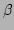Next: Introduction

### Abstract:

The dispersive interacting waves in Fermi-Pasta-Ulam (FPU) chains of particles in thermal equilibrium are studied from both statistical and wave resonance perspectives. It is shown that, even in a strongly nonlinear regime, the chain in thermal equilibrium can be effectively described by a system of weakly interacting renormalized nonlinear waves that possess (i) the Rayleigh-Jeans distribution and (ii) zero correlations between waves, just as noninteracting free waves would. This renormalization is achieved through a set of canonical transformations. The renormalized linear dispersion of these renormalized waves is obtained and shown to be in excellent agreement with numerical experiments. Moreover, a dynamical interpretation of the renormalization of the dispersion relation is provided via a self-consistency, mean-field argument. It turns out that this renormalization arises mainly from the trivial resonant wave interactions, i.e., interactions with no momentum exchange. Furthermore, using a multiple time-scale, statistical averaging method, we show that the interactions of near-resonant waves give rise to the broadening of the resonance peaks in the frequency spectrum of renormalized modes. The theoretical prediction for the resonance width for the thermalized-FPU chain is found to be in very good agreement with its numerically measured value.

# Interactions of renormalized waves in thermalized Fermi-Pasta-Ulam chains

Boris Gershgorintex2html_wrap_inline$^1$, Yuri V. Lvovtex2html_wrap_inline$^1$ and David Caitex2html_wrap_inline$^2$,

tex2html_wrap_inline$^1$ Department of Mathematical Sciences, Rensselaer Polytechnic Institute, Troy, NY 12180
tex2html_wrap_inline$^2$ Courant Institute of Mathematical Sciences, New York University, New York, NY 10012Next: Introduction
Dr Yuri V Lvov 2007-04-11